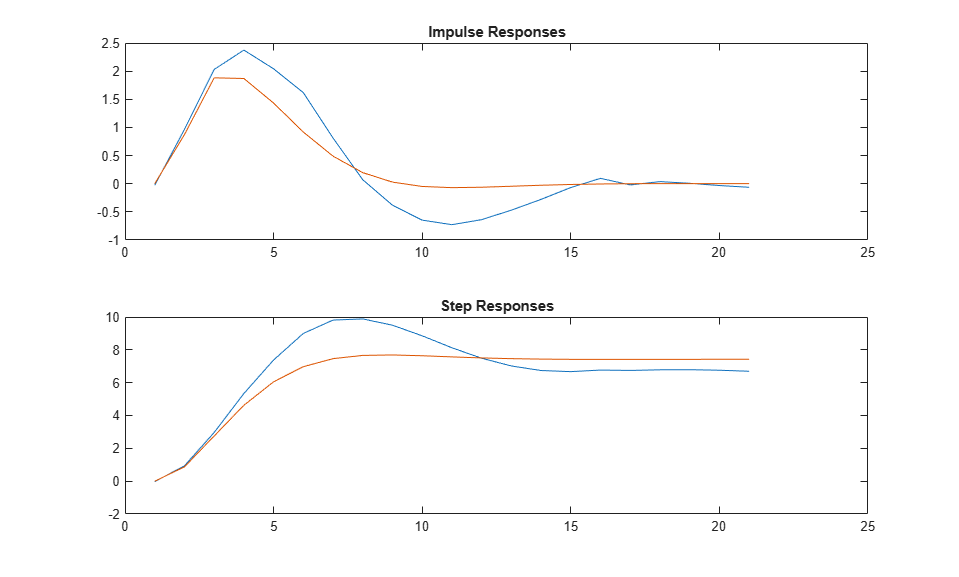Main Content

# cra

Estimate impulse response using prewhitened-based correlation analysis

## Syntax

```ir=cra(data) [ir,R,cl] = cra(data,M,na,plot) ```

## Description

`ir=cra(data)` estimates the impulse response for the time-domain data, `data`.

```[ir,R,cl] = cra(data,M,na,plot)``` estimates correlation/covariance information, `R`, and the 99% confidence level for the impulse response, `cl`.

The `cra` command first computes an autoregressive model for the input u as $A\left(q\right)u\left(t\right)=e\left(t\right)$, where e is uncorrelated (white) noise, q is the time-shift operator, and A(q) is a polynomial of order `na`. The command then filters u and output data y with A(q) to obtain the prewhitened data. The command then computes and plots the covariance functions of the prewhitened y and u and the cross-correlation function between them. Positive values of the lag variable then correspond to an influence from u to later values of y. In other words, significant correlation for negative lags is an indication of feedback from y to u in the data. A properly scaled version of this correlation function is also an estimate of the system impulse response. This is also plotted along with 99% confidence levels. The output argument `ir` is this impulse response estimate, so that its first entry corresponds to lag zero. (Negative lags are excluded in `ir`.) In the plot, the impulse response is scaled so that it corresponds to an impulse of height 1`/`T and duration T, where T is the sample time of the data.

## Input Arguments

 `data` Input-output data. Specify `data` as an `iddata` object containing time-domain data only. `data` should contain data for a single-input, single-output experiment. For the multivariate case, apply `cra` to two signals at a time, or use `impulse`. `M` Number of lags for which the covariance/correlation functions are computed. `M` specifies the number of lags for which the covariance/correlation functions are computed. These are from `-M` to `M`, so that the length of `R` is `2M+1`. The impulse response is computed from `0` to `M`. Default: 20 `na` Order of the AR model to which the input is fitted. For the prewhitening, the input is fitted to an AR model of order `na`. Use `na = 0` to obtain the covariance and correlation functions of the original data sequences. Default: 10 `plot` Plot display control. Specify plot as one of the following integers: 0 — No plots are displayed.1 — Plots the estimated impulse response with a 99% confidence region.2 — Plots all the covariance functions. Default: 1

## Output Arguments

 `ir` Estimated impulse response. The first entry of `ir` corresponds to lag zero. (Negative lags are excluded in `ir`.) `R` Covariance/correlation information. The first column of `R` contains the lag indices. The second column contains the covariance function of the (possibly filtered) output. The third column contains the covariance function of the (possibly prewhitened) input.The fourth column contains the correlation function. The plots can be redisplayed by `cra(R)`. `cl` 99 % significance level for the impulse response.

## Examples

collapse all

Compare a second-order ARX model's impulse response with the one obtained by correlation analysis.

```load iddata1 z = z1; ir = cra(z); m = arx(z,[2 2 1]); imp = [1;zeros(20,1)]; irth = sim(m,imp); subplot(211) plot([ir irth]) title('Impulse Responses') subplot(212) plot([cumsum(ir),cumsum(irth)]) title('Step Responses') ```## Alternatives

An often better alternative to `cra` is `impulseest`, which use a high-order FIR model to estimate the impulse response.

## See Also

Introduced before R2006a

## Support평가판 신청